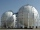A domed stadium is in the shape of spherical segment with a base radius of 150 m. The dome must contain a volume of 3500000 m³. Determine the height of the dome at its centre to the nearest tenth of a meter.

Result

x =  88.7 m

Solution:Equation is non-linear.h1 = 88.693342641435
h2 = -44.34667132+270.92412549i
h3 = -44.34667132-270.92412549i

Calculated by our simple equation calculator.

Leave us a comment of example and its solution (i.e. if it is still somewhat unclear...):Be the first to comment!To solve this example are needed these knowledge from mathematics:

Looking for help with calculating roots of a quadratic equation? Do you have a linear equation or system of equations and looking for its solution? Or do you have quadratic equation? Try our complex numbers calculator. Tip: Our volume units converter will help you with converion of volume units. Pythagorean theorem is the base for the right triangle calculator.

Next similar examples:

1. Swimming poolThe pool shape of cuboid is 299 m3 full of water. Determine the dimensions of its bottom if water depth is 282 cm and one bottom dimension is 4.7 m greater than the second.
2. Hemispherical hollowThe vessel hemispherical hollow is filled with water to a height of 10 cm =. How many liters of water are inside if the inside diameter of the hollow is d = 28cm?
3. Spherical tankThe tank of a water tower is a sphere of radius 35ft. If the tank is filled to one quarter of full, what is the height of the water?
4. Theorem proveWe want to prove the sentence: If the natural number n is divisible by six, then n is divisible by three. From what assumption we started?
5. Linear imaginary equationGiven that ? "this is z star" Find the value of the complex number z.
6. EquationEquation ? has one root x1 = 8. Determine the coefficient b and the second root x2.Find the roots of the quadratic equation: 3x2-4x + (-4) = 0.
8. RootsDetermine the quadratic equation absolute coefficient q, that the equation has a real double root and the root x calculate: ?
9. Complex number coordinatesWhich coordinates show the location of -2+3i
10. Solve 3Solve quadratic equation: (6n+1) (4n-1) = 3n2
11. DiscriminantDetermine the discriminant of the equation: ?
12. CathetiThe hypotenuse of a right triangle is 41 and the sum of legs is 49. Calculate the length of its legs.
13. ABS CNCalculate the absolute value of complex number -15-29i.Quadratic equation ? has roots x1 = 80 and x2 = 78. Calculate the coefficients b and c.If 5x + x² > 100, then x is notCalculate height and volume of a regular tetrahedron whose edge has a length 18 cm.If a2-3a+1=0, find (i)a2+1/a2 (ii) a3+1/a3